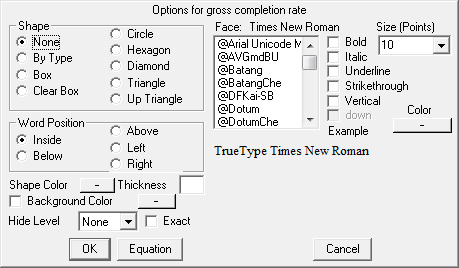# Vensim Help

 Navigation: Reference Guide > Equation Editor Starting and Closing the Equation Editor

There are several ways to access the Equation Editor from the Sketch Editor.

1        When you select the Equations tool in the Sketch Editor, clicking on a variable will open the equation for that variable.

2        When any other tool is selected, holding down the Shift and Ctrl keys and clicking (or holding down the Shift key and right-clicking) on a variable will also open the Equation Editor on that variable.

3        Right-click on the variable to display the Variable Options dialog.  Then select the equation button:4        You can have an Equation Edit tool in the analysis toolset.  When this tool is invoked the Equation Editor will be opened on the Workbench Variable.  This is most useful for units checking which requires looking at the equations for a number of different variables.

5        Finally when you check the model (Model>Check Model) or attempt to simulate the model and Vensim finds an error, if you ask to correct errors the Equation Editor will open.

Dialog Operations

When the Equation Editor is open, you will not be able to perform operations outside of the Equation Editor until you close it.  If equations are created that are inconsistent with the sketch, Vensim will automatically update the sketch to keep it consistent.

Resizing the Equation Editor

You can resize the Equation Editor by dragging on the lower right-hand corner (though you cannot make is smaller than its initial size).   This will change the size of the editing window for equations and can be useful if you have a big screen and a model with long equations. The size, position and layout of the equation editor is persistent across Vensim sessions.

Closing the Equation Editor

Whenever you close the Equation Editor, Vensim will try to make sense of what you have entered.  If the model has problems, these will be marked, and whatever causal structure can be interpreted will be interpreted.  In this manner it is always possible to do causal tracing, though for subscripted variables the accuracy of the causal tracing can be limited.

When you use the Equation Editor to make a change to the structure of the model Vensim will check to be sure that the sketch information for the model is also updated.  If, for example you remove a feedback link, Vensim will remove that feedback link in any views it may have appeared.  When you close the Equation Editor the Sketch Editor will sometimes flash because of this updating.  This can happen independent of whether you launch the Equation Editor with a Sketch tool or by clicking on an Equation Editor tool on the Analysis toolset